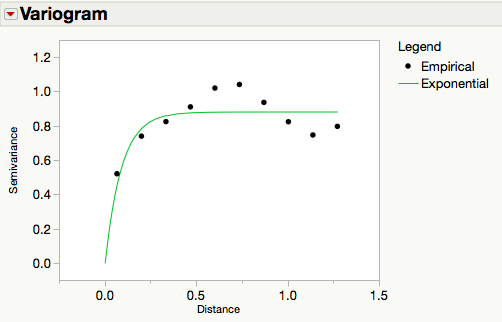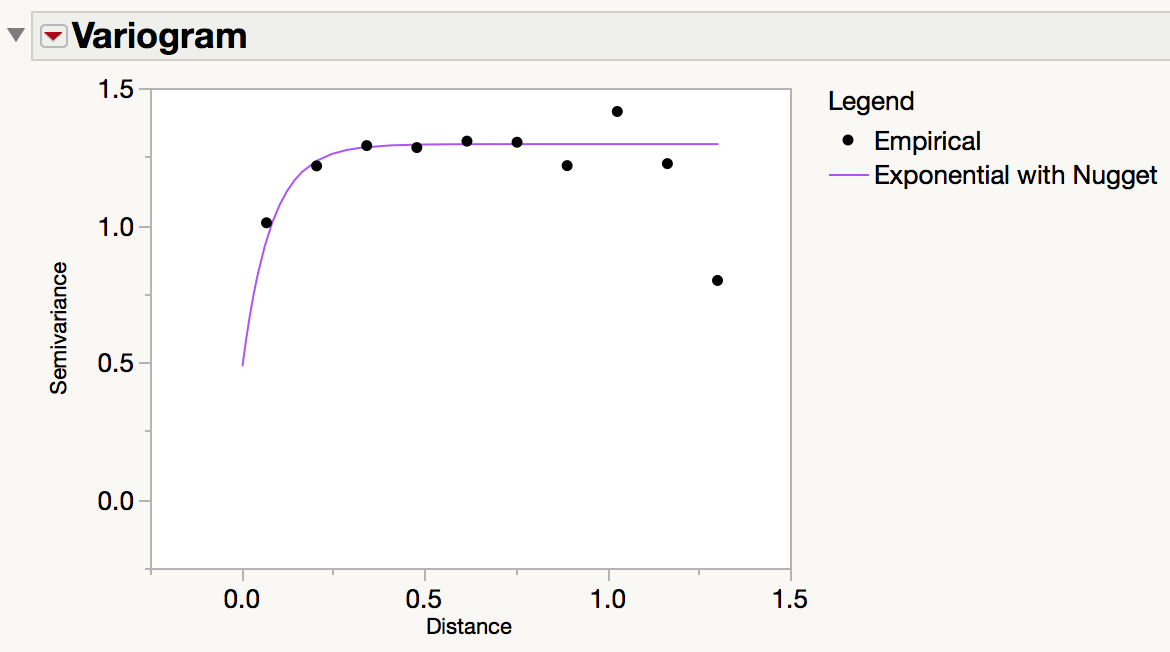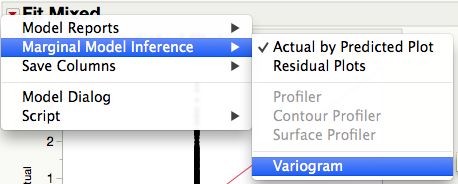Our World Statistics Day conversations have been a great reminder of how much statistics can inform our lives. Do you have an example of how statistics has made a difference in your life? Share your story with the Community!
Choose Language Hide Translation Bar
Coming in JMP Pro 12: Variograms in Fit Mixed

In JMP Pro 11, we introduced the Fit Mixed platform for fitting models with a variety of covariance structures and random effects. With JMP Pro 12, we have improved on this platform, with noticeable changes coming to models with spatial covariance structures. These changes are detailed below.

## Enhanced speed

Fitting models with a spatial covariance requires a high computing cost, particularly as the number of observations increases. For JMP Pro 12, the algorithm we use to fit models in the Fit Mixed platform has been improved by Chris Gotwalt, the Director of Statistical R&D at JMP. His work further reduced the number of computations required to fit all models in Fit Mixed. This has greatly reduced the time required to fit spatial models in particular.

In our testing, we have experienced anywhere from a 5 to 15 times increased speed when fitting these spatial models. In practical terms, this means we have reduced fits that took more than an hour to complete before to now being completed in just over 10 minutes!

## Variograms

We have also added variograms in JMP Pro 12, a visual tool to examine and diagnose the existence of spatial correlation. The variogram is available when you fit an isotropic spatial model (an AR(1) or one of Power, Exponential, Gaussian, or Spherical with or without a nugget). The variogram below visualizes the change in covariance as observation locations move apart in time or space.The variogram plots the semivariance (half the variance) of the difference between observations at two locations versus the distance between the locations. As depicted in the variogram above, we can see that observations close together have strong correlation, hence a small semivariance.

This correlation decreases as distance increases, leading the semivariance to increase. The semivariance no longer increases at what is known as the range, the distance at which observations have little to no correlation. In the variogram above, this is approximately at a distance of 0.25.

The maximum value of the semivariance is known as the sill, and in most cases with the models fit in JMP Pro, this is simply the variance of the observations. In the presence of non-spatial error, a nugget can be included to estimate this variability. The nugget effect is depicted in the variogram below.The semivariance is zero at distance zero, but the semivariance then jumps up to the nugget as soon as the distance between locations is positive. In the variogram above, the nugget is approximately 0.5.

### Residual-only models

The variogram is also available when you fit a residual-only model. This option is accessed under Marginal Model Inference:After selecting this option and specifying the column(s) to use for computing distance in time or space, the empirical variogram is shown. The variogram red triangle menu allows you to fit one or more spatial models to this empirical variogram.

In this case, the variogram can be used to determine if a spatial covariance should be added to the residual only model. Under the absence of spatial correlation, the empirical variogram would likely appear flat, suggesting no change in correlation over distance.

Above, we see that there appears to be an increase in the empirical variogram as distance increases, and one of the Exponential, Gaussian, or Spherical models may be suitable (the Power model does not appear to fit the empirical variogram well). After using Fit Mixed to formally fit a model to each one of these correlation structures, we find that the Exponential model is preferred because it has the smallest corrected Akaike Information Criterion (AICc) and Bayesian Information Criterion (BIC). This means that the variogram should be used to identify possible models that can then be formally fit with Fit Mixed. With these fits, an AICc/BIC comparison should be used to choose the best model.

We hope you will find the enhanced speed and variogram addition useful, and we look forward to any questions or feedback you have in the comments!

Article Labels

There are no labels assigned to this post.

Article Tags